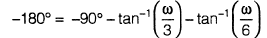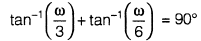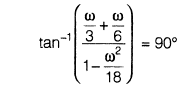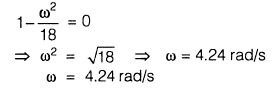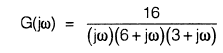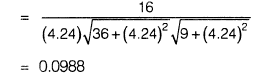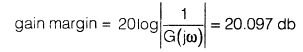Courses

# Control System - MCQ Test 1

## 10 Questions MCQ Test Mock Test Series for Electrical Engineering (EE) GATE 2020 | Control System - MCQ Test 1

Description
This mock test of Control System - MCQ Test 1 for GATE helps you for every GATE entrance exam. This contains 10 Multiple Choice Questions for GATE Control System - MCQ Test 1 (mcq) to study with solutions a complete question bank. The solved questions answers in this Control System - MCQ Test 1 quiz give you a good mix of easy questions and tough questions. GATE students definitely take this Control System - MCQ Test 1 exercise for a better result in the exam. You can find other Control System - MCQ Test 1 extra questions, long questions & short questions for GATE on EduRev as well by searching above.
QUESTION: 1

### A system has bandwidth as 4000 Hz with rise time 2.5 seconds. If the rise time decreased to 1.25 seconds then the bandwidth is

Solution: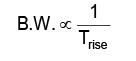QUESTION: 2

Solution:
QUESTION: 3

### The purpose of PI controller is to improve _____________ of the system.

Solution:
QUESTION: 4

The current locus of the circuit shown in below figure is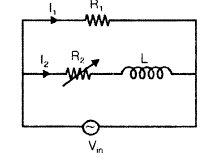Solution:
QUESTION: 5

The step response of an under damped system is given as [1 − Ae−Bt sin(8t + 53.13°)]. The value of ‘A’ & ‘B’ respectively are

Solution: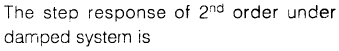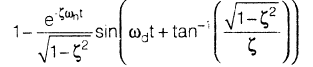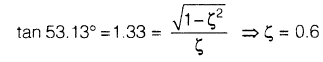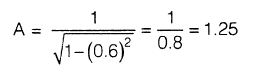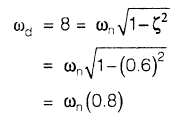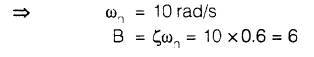QUESTION: 6

The settling time required to the system which has transfer  function as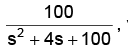with 5% tolerance is

Solution: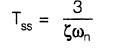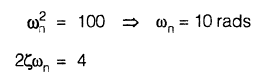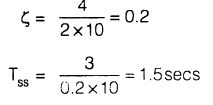QUESTION: 7

The ‘root locus’ of a system is shown in the below figure. Its transfer function is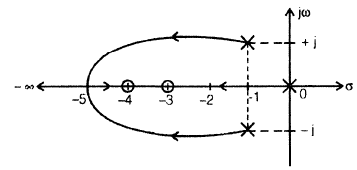Solution: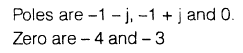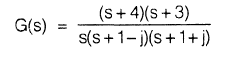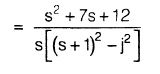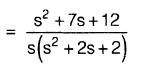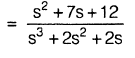QUESTION: 8

Consider the circuit shown in the below figure: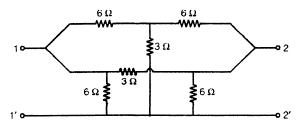Q.

The s.c. input admittance of the above network is

Solution: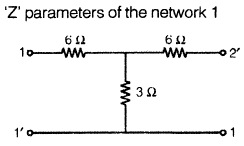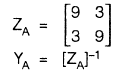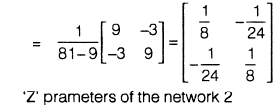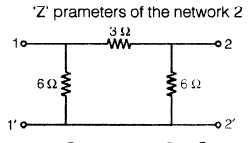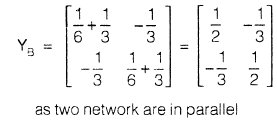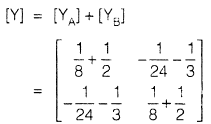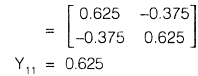QUESTION: 9

Consider the circuit shown in the below figure:Q.

The o.c. output impedance of the above network is

Solution: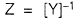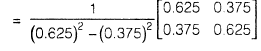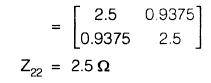QUESTION: 10

The gain margin of the system which has transfer function as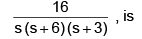Solution: